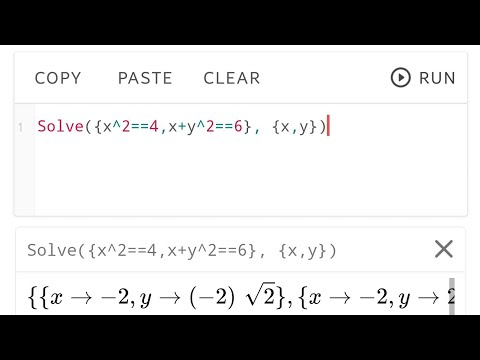# Calculator N+ - Math Solver - CAS calculatorEveryone
12,696The Calculator provides the following features:
ALGEBRA
1. Computer science
2. Solving the equation:
3. Solve system equations
4. Graphing
5. Cartesian geometry sand(Viet Nam Education)
6. Unit Conversions
8. Simplify expressions
9. Polynomial factorization.
10. Binomial expansion Newton
11. Matrix: evalute matrix step by step

ANALYTICS
1. Derivative
2. Antiderivative
3. Definite integrals
4. Find the limit of the sequence, function

TRIGONOMETRIC
1, Trigonometric expand: sin(2x) -> 2sin(x)cos(x)
2. Trigonometric reduce: 2sin(x)cos(x) -> sin(2x)
3. Trigonometric to exponent: sinh(x) -> (e^x-e^(-x))/2

DETERMINATION OF STATISTICS
1. Combination
2. Permutation

SOME OTHER FEATURES
1. Prime factors
2. Modulo
3. Catalan number
4. Fibonacci number

The Calculator does not show the steps to solve the equations, system of equations, derivative... but only for the final result.
Enter sin(30°) to calculate sine Degree mode

The computer can work in two modes:
- Decimal mode: 0.12312312323
- Mode fractions: for results with arbitrary precision, for example 9^99999

Any suggestions on the application please send mail: Tranleduy1233@gmail.com.

See in github https://github.com/tranleduy2000/ncalc
Collapse

## Reviews

Review policy and info
4.6
12,696 total
5
4
3
2
1

## What's New

Bug fixes
Update math library
Collapse

Updated
November 24, 2019
Size
14M
Installs
500,000+
Current Version
3.4.9
Requires Android
4.1 and up
Content Rating
Everyone
Permissions
Offered By
Mr Duy
Developer
Long Xuyen city, An Giang province, Viet Nam